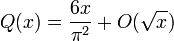# BZOJ2440: [中山市选2011]完全平方数(莫比乌斯+容斥原理)

2018/03/06 20:44

## 2440: [中山市选2011]完全平方数

Time Limit: 10 Sec  Memory Limit: 128 MB
Submit: 4920  Solved: 2389
[Submit][Status][Discuss]

4
1
13
100
1234567

1
19
163
2030745

,    T ≤ 50

## Source

$mid - (A_{p_1} + A_{p_2} + \cdots + A_{p_k}) + (A_{p_1 \cdot p_2} + A_{p_1 \cdot p_3} + \cdots + A_{p_{k-1} \cdot p_k}) + \cdots + (-1)^{k} A_{\prod_{i=1}^{k} p_i}$#include<cstdio>
#include<cstring>
#include<cmath>
#define int long long
using namespace std;
const int MAXN=1e6+10;
{
char c=getchar();int x=0,f=1;
while(c<'0'||c>'9'){if(c=='-')f=-1;c=getchar();}
while(c>='0'&&c<='9'){x=x*10+c-'0';c=getchar();}
return x*f;
}
int N;
int vis[MAXN],prime[MAXN],mu[MAXN],tot=0;
void GetMu()
{
vis=1;mu=1;
for(int i=2;i<=N;i++)
{
if(!vis[i]) prime[++tot]=i,mu[i]=-1;
for(int j=1;i*prime[j]<=N&&j<=tot;j++)
{
vis[i*prime[j]]=1;
if(i%prime[j]==0){mu[i*prime[j]]=0;break;}
else mu[i*prime[j]]=-mu[i];
}
}
}
int check(int val)
{
int limit=sqrt(val),ans=0;
for(int i=1;i<=limit;i++)
ans+=mu[i]*(val/(i*i));
return ans;
}
main()
{
#ifdef WIN32
freopen("a.in","r",stdin);
#else
#endif
N=1e6+10;
GetMu();
while(QWQ--)
{
int l=1,r=x<<1,ans=0;
while(l<=r)
{
int mid=l+r>>1;
if(check(mid)>=x) ans=mid,r=mid-1;
else l=mid+1;
}
printf("%d\n",ans);
}
return 0;
} 

0
0 收藏

### 作者的其它热门文章0 评论
0 收藏
0